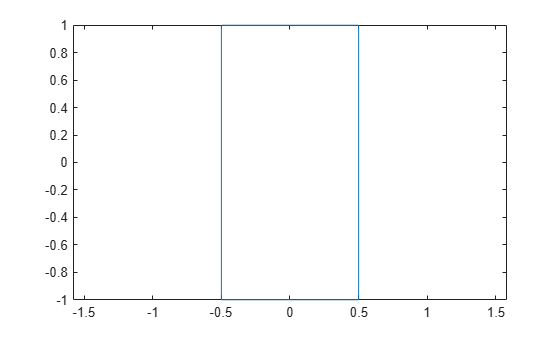# plot

Plot boundary of shape

## Syntax

``p = plot(shape,varargin)``

## Description

example

````p = plot(shape,varargin)` plots the boundary of the shape and returns the line handle.```

## Examples

collapse all

Create a rectangular shape and plot it.

```r = antenna.Rectangle; p = plot(r);```## Input Arguments

collapse all

Shape created using custom elements and shape objects of Antenna Toolbox, specified as an object handle.

Example: `plot(rectangle)` where rectangle is created using `antenna.Rectangle` object.